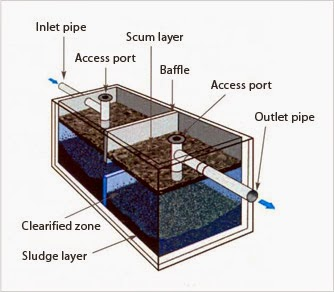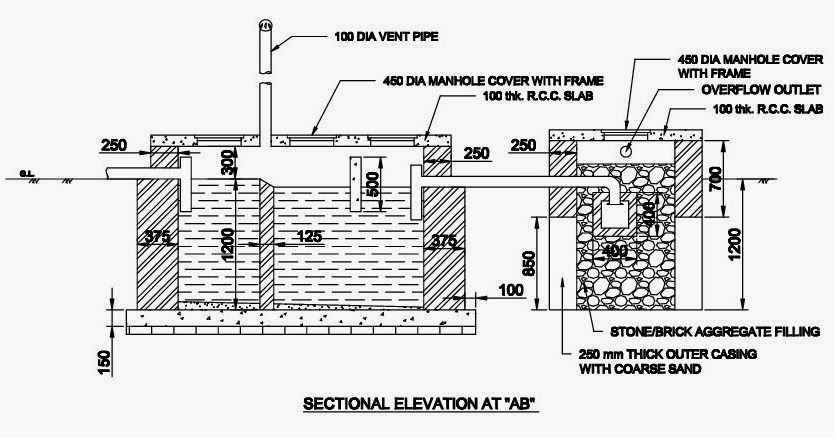# Septic Tank Design Easily 5 Minutes

Last Updated on

Septic System Design or Septic Tanks Design can be done in various process, but most of them are critical and time taking laborious process. Here in this article I have given tutorial on how to design Septic Tank Easily within 5 minutes and Determine Septic Tank Size. Here I will also discuss some about the general Septic Tank Construction for designing of septic tank successfully you have to follow the steps given below one by one

### Step 1: Calculation of Numbers of User :

In any septic system design this is a very very important step as it will determine the total capacity of the septic tank required.This is the total numbers of user who will be using the septic tank within a single day. For the calculation purpose we must consider the maximum practical numbers of user who may use the W.C. within a day. The calculation can be done very easily, like suppose there is a 4 storied building and having two families on each floor. And each family contains on an average of 5 person. Therefore the building is accommodating (2 x 4) = 8 families and again 8 families will equal to users of (8 x 5) = 40 Nos. Users. Generally in no case user number is kept less than 20 users. Therefore here we will design a septic tank for 40 UsersDIFFERENT PARTS OF A SEPTIC TANK

### Step 2: Calculation of Liquid Volume in Septic Tank :

In this step we will calculate the volume of waste liquid in septic tank which will occurs due to the use of 40 user. For normal detention period It is considered in designing purpose that a septic tank requires 0.085 cu.m. of space for waste liquid of each person per day is sufficient for design, keeping in mind septic tank cleaning which will be done periodically. also a future provision should be provided, which in general is considered as 25% of the actual volume required.
Therefore, Volume of Liquid @ 0.085 cu.m. per capita per day = 40×0.085 = 3.40 cu.m.
Allowing a future provision of 25% extra, therefore Total Volume of Liquid = 3.40+ 25% = 4.25 cu.m.

### Step 3: Calculation of Internal Dimension Of Septic Tank :

Now we have to find out the internal depth, length and breadth of the septic tank. first of all we have to consider a liquid waste depth suitably from 1m to 2m for better efficiency. if the waste volume is large then we need to consider greater depth for making the length and breadth smaller to accommodate within the land. then as we know volume = length x breadth x depth (height), there for as we have already assumed the liquid depth so we can find the length and breadth by calculating the surface area of the liquid surface by dividing the waste volume with liquid depth
Now, considering a Liquid Depth of 1.20m.
Therefore, Surface Area of Liquid = (4.25/1.20) = 3.54 sq.m.
so now this 3.54 sq.m. = Length x Breadth. Now we can calculate the length and the breadth easily by either adopting a suitable breadth according to the land available or according to some proportions like considering the length of septic tank as the twice of its breadth, L=2B etc.
so we will have Area = Length x Breadth
or 3.54 = L x B
or 3.54 = 2B x B
or 2B^2 = 3.54
or B = Root of (3.54/2) = 1.33m say 1.40 m
Therefore, L = 2B = 2 x 1.40 = 2.80m, But more accurately the length shall be calculated like the following one, here L x B = 3.54 , now as we know the value of B therefore we can obtain the the value of Length by:
L x B = 3.54
or L x 1.40 = 3.54
or L = (3.54/1.40) = 2.53 m say 2.60m.
But there is another process which is suitable where there breadth is restricted, in those cases we have to limit the breadth by adopting a value, and then calculate the rest by the following :
Now, considering the Breadth of Septic Tank as 1.20m

Therefore,
Length x Breadth = 3.54 sq.m.
Or Length = (3.54/Breadth) = (3.54/1.20) = 2.95m , say 3m
Now this dimensions are must, but apart from this we have to provide a clear space above the liquid waste in septic tank so that the gas evolving from it can freely move and can escape through the vent pipe. For this reason we consider a gap above the liquid or free board of sufficient space, generally like the following:
Considering a Free Board over the Liquid surface of 300mm.
Therefore, Total Internal Depth of the Septic Tank will be = 1.20 + 0.30 = 1.50m.

Therefore, the Internal Dimensions of the Septic Tank are as follows :

Length = 2.60m      Breadth = 1.40 m    Height = 1.50m

Now prepare neat drawing of the above, below I have provided one, but the dimensions will not tally with the above one, it is just for reference.SEPTIC TANK SECTIONAL ELEVATION WITH SOAK PIT

Keep visiting MyCivil – Civil Engineering Redefined for ore similar interesting Articles. Do Comment in this post and share it in your network if possible to help Techno Genome Grow.

If you like this Article then I am sure you will like the following well researched articles too, come on check them out also

### Come Connect with me On Social Media

#### One Response

1.Paige Smith February 21, 2016